# How to Calculate Arc Length of a Circle, Segment and Sector Area

Eugene is a qualified control/instrumentation engineer Bsc (Eng) and has worked as a developer of electronics & software for SCADA systems.

In this tutorial you'll learn about:

• names for different parts of a circle
• degrees and radians and how to convert between them
• chords, arcs and secants
• sine and cosine
• how to work out the length of an arc and chord
• how to calculate the area of sectors and segments
• the equation of a circle in the Cartesian coordinate system

## What Is a Circle?

"A locus is a curve or other figure formed by all the points satisfying a particular equation."

A circle is a single-sided shape, but it can also be described as a locus of points where each point is equidistant (the same distance) from the centre.

## Angle Formed by Two Rays Emanating From the Center of a Circle

An angle is formed when two lines or rays that are joined together at their endpoints, diverge or spread apart. Angles range from 0 to 360 degrees. We often "borrow" letters from the Greek alphabet to use in math and science. So for instance, we use the Greek letter "p" which is π (pi) and pronounced "pie" to represent the ratio of the circumference of a circle to the diameter. We also use the Greek letter θ (theta) and pronounced "the - ta", for representing angles.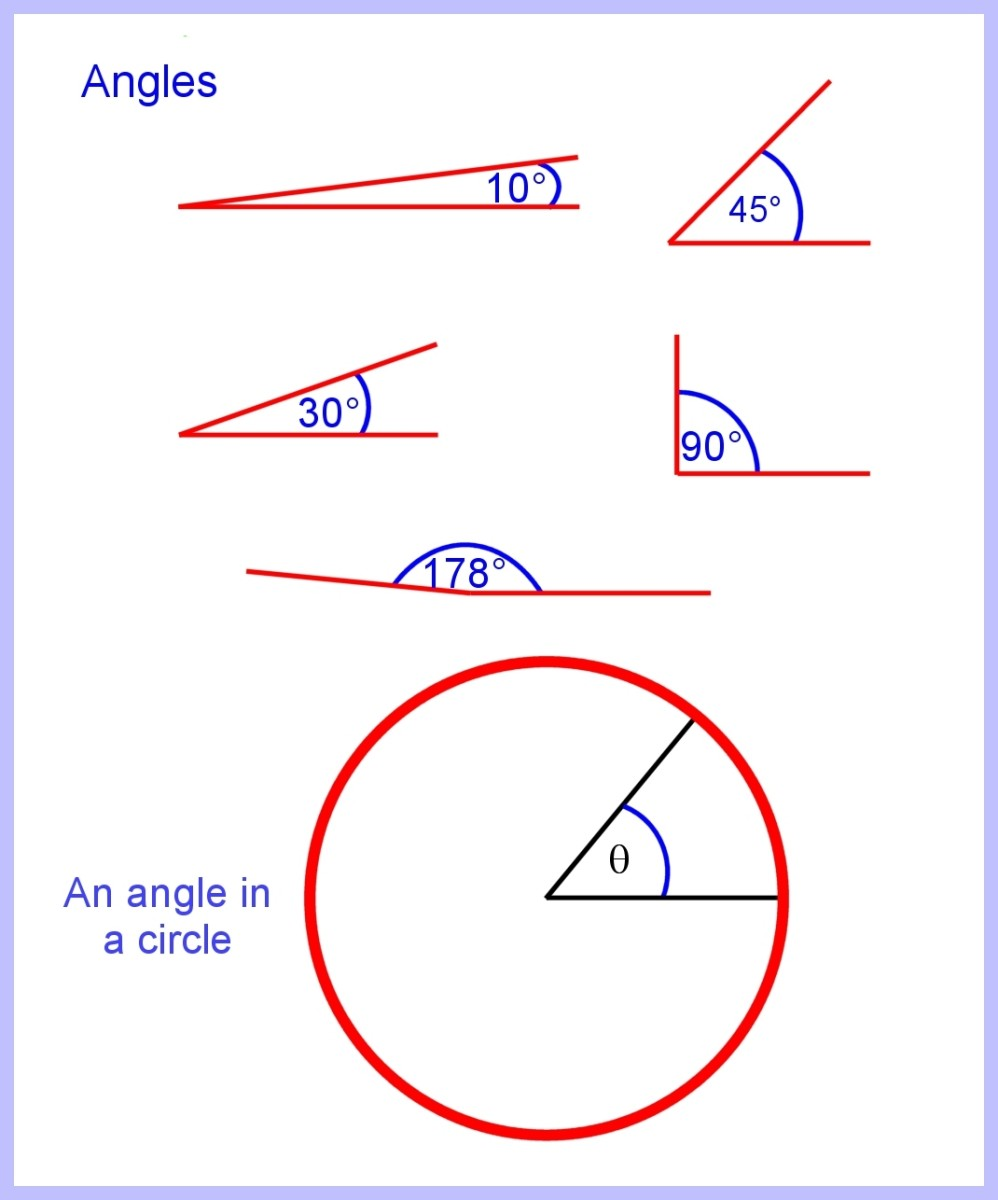An angle is formed by two rays diverging from the centre of a circle. This angle ranges from 0 to 360 degreesImage © Eugene Brennan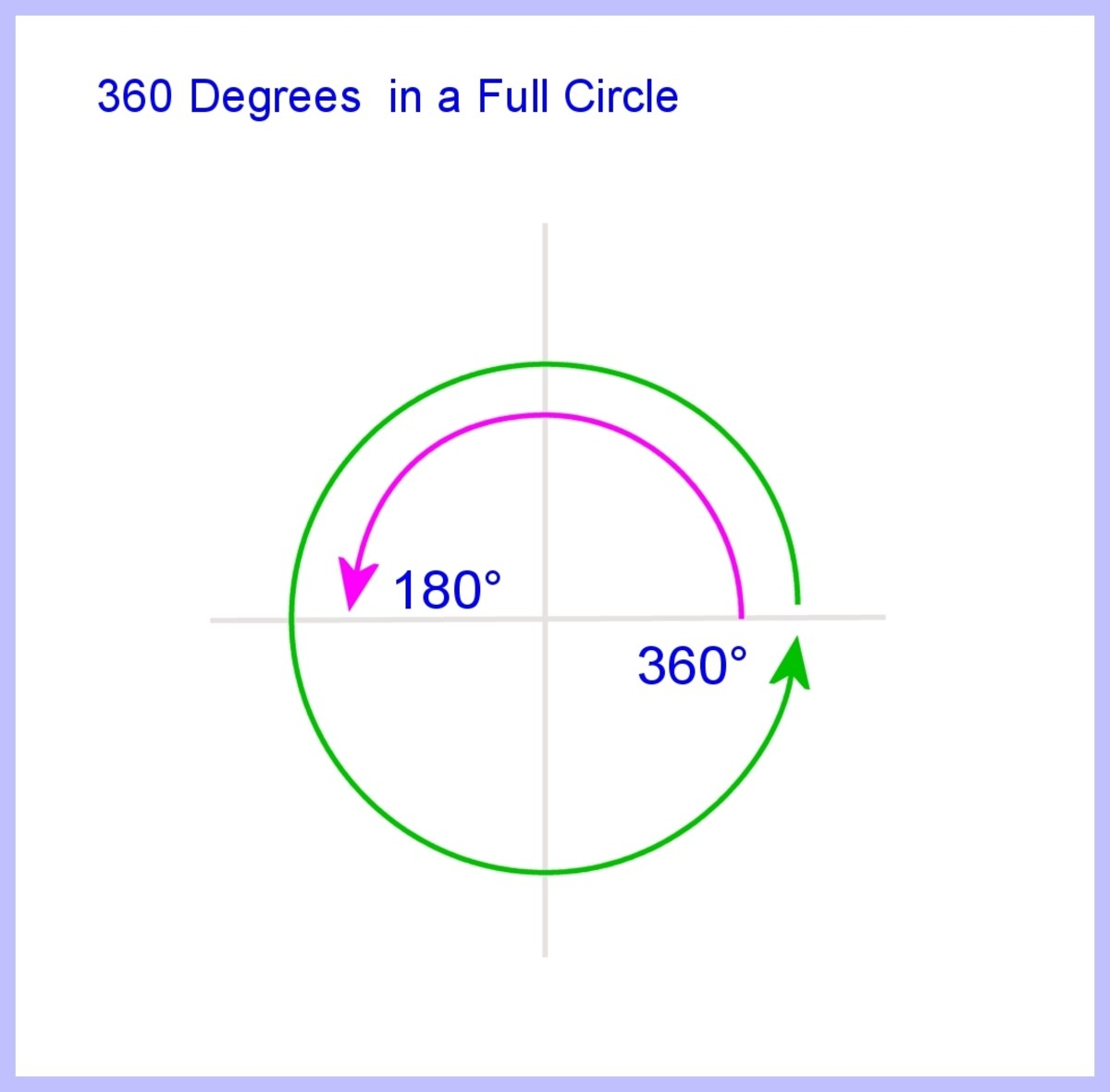360 degrees in a full circleImage © Eugene Brennan

## Parts of a Circle

• A sector is a portion of a circular disk enclosed by two rays and an arc.
• A segment is a portion of a circular disk enclosed by an arc and a chord.
• A semi-circle is a special case of a segment, formed when the chord equals the length of the diameter.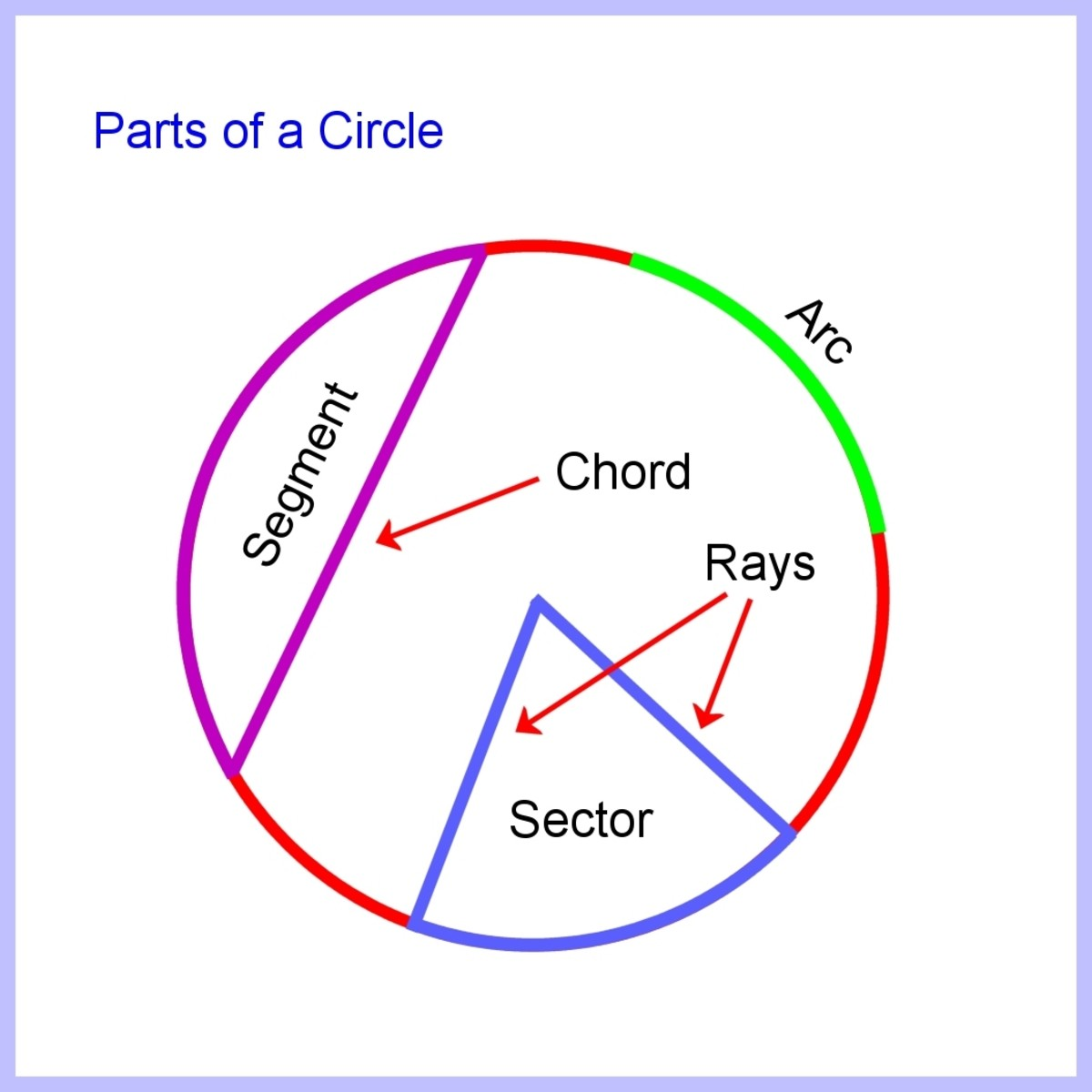Arc, sector, segment, rays and chordImage © Eugene Brennan

## What Is Pi (π) ?

Pi represented by the Greek letter π is the ratio of the circumference to the diameter of a circle. It's a non-rational number which means that it can't be expressed as a fraction in the form a/b where a and b are integers.

Pi is equal to 3.1416 rounded to 4 decimal places.

## What's the Length of the Circumference of a Circle?

If the diameter of a circle is D and the radius is R.

Then the circumference C = πD

But D = 2R

Scroll to Continue

## Read More From Owlcation

So in terms of the radius R

C = πD = 2πR

## What's the Area of a Circle?

The area of a circle is A = πR2

But R = D/2

So the area in terms of the radius R is

A = πR2 = π (D/2)2 = πD2/4

## What Are Degrees and Radians?

Angles are measured in degrees, but sometimes to make the mathematics simpler and elegant it's better to use radians which is another way of denoting an angle. A radian is the angle subtended by an arc of length equal to the radius of the circle. ( "Subtended" means produced by joining two lines from the end points of the arc to the center).

An arc of length R where R is the radius of a circle, corresponds to an angle of 1 radian.

So if the circumference of a circle is 2πR i.e 2π times R, the angle for a full circle will be 2π times 1 radian or 2π radians.

And 360 degrees = 2π radians.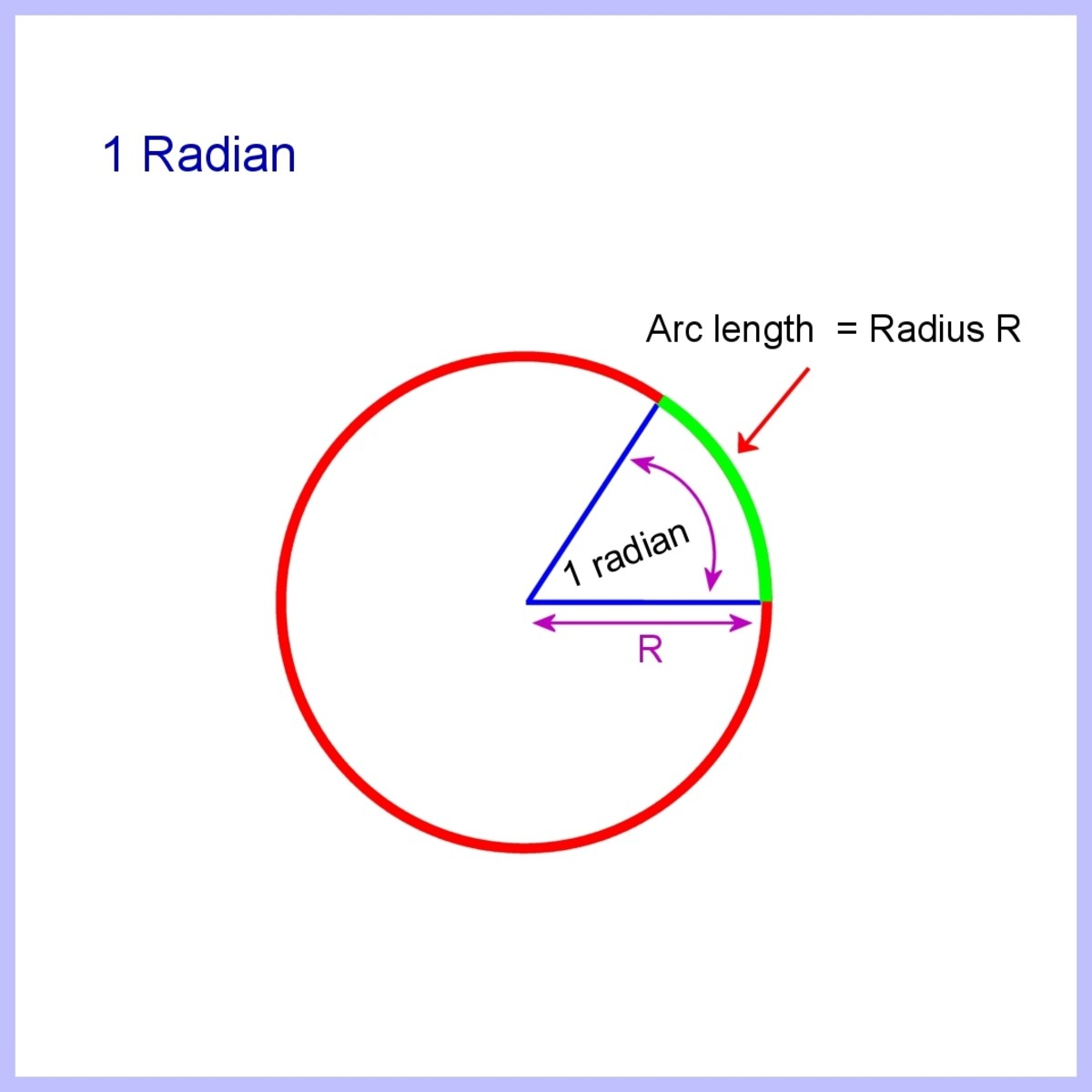A radian is the angle subtended by an arc of length equal to the radius of a circle.Image © Eugene Brennan

## How to Convert From Degrees to Radians

1. 360 degrees = 2π radians
2. Dividing both sides by 360 gives
3. 1 degree = 2π /360 radians
4. Then multiply both sides by θ
5. θ degrees = (2π/360) x θ = θ(π/180) radians
6. So to convert from degrees to radians, multiply by π/180

## How to Convert From Radians to Degrees

1. 2π radians = 360 degrees
2. Divide both sides by 2π giving
3. 1 radian = 360 / (2π) degrees
4. Multiply both sides by θ, so for an angle θ radians
5. θ radians = 360/(2π) x θ = (180/π)θ degrees
6. So to convert radians to degrees, multiply by 180/π

## How to Find the Length of an Arc

You can work out the length of an arc by calculating what fraction the angle is of the 360 degrees for a full circle.

1. A full 360 degree angle has an associated arc length equal to the circumference C
2. So 360 degrees corresponds to an arc length C = 2πR
3. Divide by 360 to find the arc length for one degree:
4. 1 degree corresponds to an arc length 2πR/360
5. To find the arc length for an angle θ, multiply the result above by θ:
6. 1 x θ = θ corresponds to an arc length (2πR/360) x θ

So arc length s for an angle θ is:

s = (2πR/360) x θ = πRθ/180

The derivation is much simpler for radians:

By definition, 1 radian corresponds to an arc length R

So if the angle is θ radians, multiplying by θ gives:

Arc length s = R x θ =

## What Are Sine and Cosine?

A right-angled triangle has one angle measuring 90 degrees. The side opposite this angle is known as the hypotenuse and it is the longest side. Sine and cosine are trigonometric functions of an angle and are the ratios of the lengths of the other two sides to the hypotenuse of a right-angled triangle.

In the diagram below, one of the angles is represented by the Greek letter θ.

The side a is known as the "opposite" side and side b is the "adjacent" side to the angle θ.

### cosine θ = length of adjacent side / length of hypotenuse

Sine and cosine apply to an angle, not necessarily an angle in a triangle, so it's possible to just have two lines meeting at a point and to evaluate sine or cos for that angle. However sine and cos are derived from the sides of an imaginary right angled triangle superimposed on the lines. In the second diagram below, you can imagine a right angled triangle superimposed on the purple triangle, from which the opposite and adjacent sides and hypotenuse can be determined.

Over the range 0 to 90 degrees, sine ranges from 0 to 1 and cos ranges from 1 to 0

Remember sine and cosine only depend on the angle, not the size of the triangle. So if the length a changes in the diagram below when the triangle changes in size, the hypotenuse c also changes in size, but the ratio of a to c remains constant.

Sine and cosine are sometimes abbreviated to sin and cos.

## How to Calculate the Area of a Sector of a Circle

The total area of a circle is πR2 corresponding to an angle of 2π radians for the full circle.

If the angle is θ, then this is θ/2π the fraction of the full angle for a circle.

So the area of the sector is this fraction multiplied by the total area of the circle

or

(θ/2π) x (πR2) = θR2/2 with θ in radians.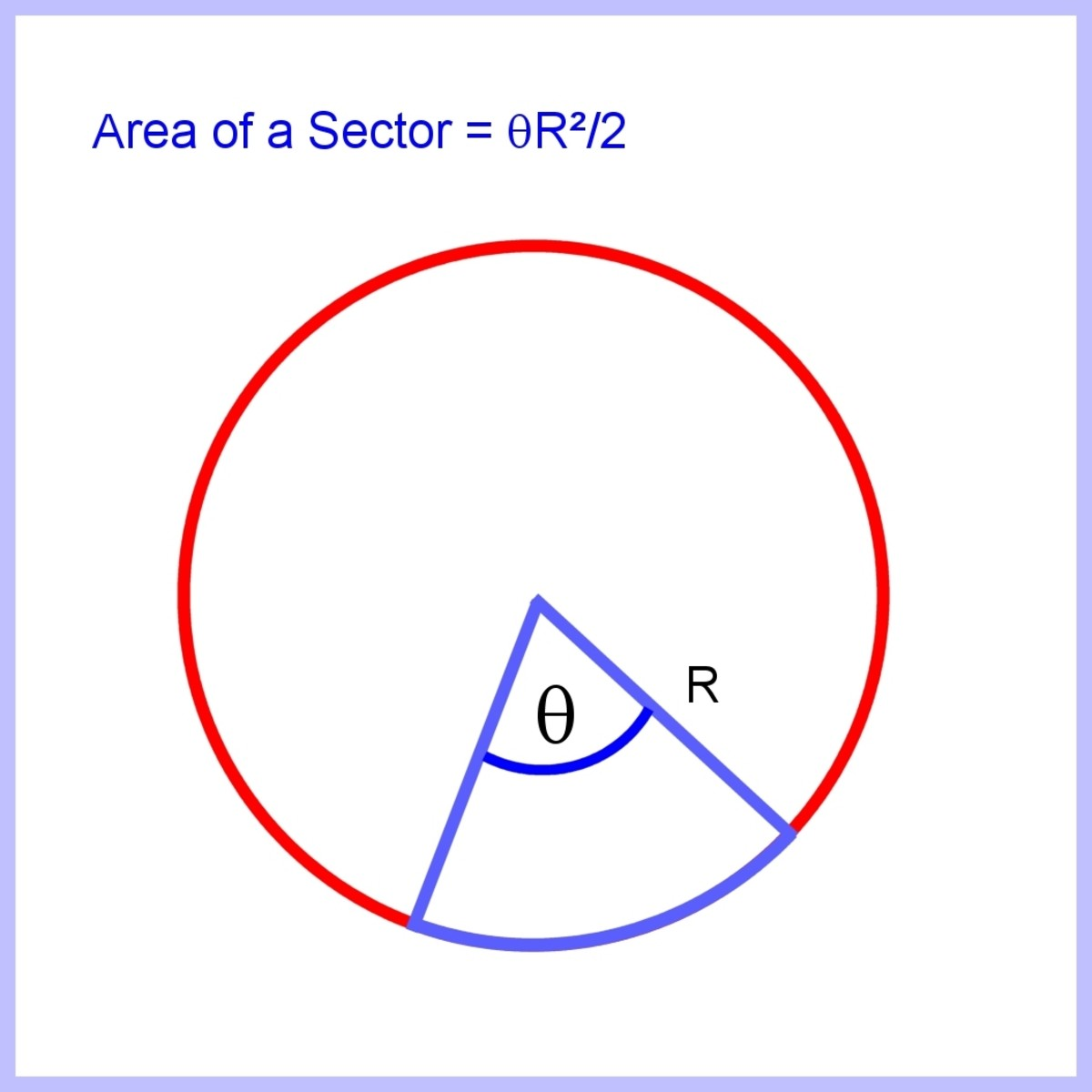Area of a sector of a circle knowing the angle θ in radiansImage © Eugene Brennan

## How to Calculate the Length of a Chord Produced by an Angle

The length of a chord can be calculated using the Cosine Rule.
For the triangle XYZ in the diagram below, the side opposite the angle θ is the chord with length c.

From the Cosine Rule:

c2 = R2 + R2 -2RRcos θ

Simplifying:

c2 = R2 + R2 -2R2cos θ

or c2 = 2R2 (1 - cos θ)

But from the half-angle formula (1- cos θ)/2 = sin 2 (θ/2) or (1- cos θ) = 2sin 2 (θ/2)

Substituting gives:

c2 = 2R2 (1 - cos θ) = 2R22sin 2 (θ/2) = 4R2sin 2 (θ/2)

Taking square roots of both sides gives:

c = 2Rsin(θ/2) with θ in radians.

A simpler derivation arrived at by splitting the triangle XYZ into 2 equal triangles and using the sine relationship between the opposite and hypotenuse, is shown in the calculation of segment area below.

## How to Calculate the Area of a Segment of a Circle

To calculate the area of a segment bounded by a chord and arc subtended by an angle θ , first work out the area of the triangle, then subtract this from the area of the sector, giving the area of the segment. (see diagrams below)

The triangle with angle θ can be bisected giving two right angled triangles with angles θ/2.

sin(θ/2) = a/R

So a = Rsin(θ/2) (cord length c = 2a = 2Rsin(θ/2)

cos(θ/2) = b/R

So b = Rcos(θ/2)

The area of the triangle XYZ is half the base by the perpendicular height so if the base is the chord XY, half the base is a and the perpendicular height is b. So the area is:

ab

Substituting for a and b gives:

Rsin(θ/2)Rcos(θ/2)

= R2sin(θ/2)cos(θ/2)

But the double angle formula states that sin(2θ) = 2sin(θ)cos(θ)

Substituting gives:

Area of the triangle XYZ = R2sin(θ/2)cos(θ/2) = R2 ((1/2)sin θ) = (1/2)R2sin θ

Also, the area of the sector is:

R2(θ/2)

And the area of the segment is the difference between the area of the sector and the triangle, so subtracting gives:

Area of segment = R2(θ/2) - (1/2)R2sin θ

= (R2/2)( θ - sin θ ) with θ in radians.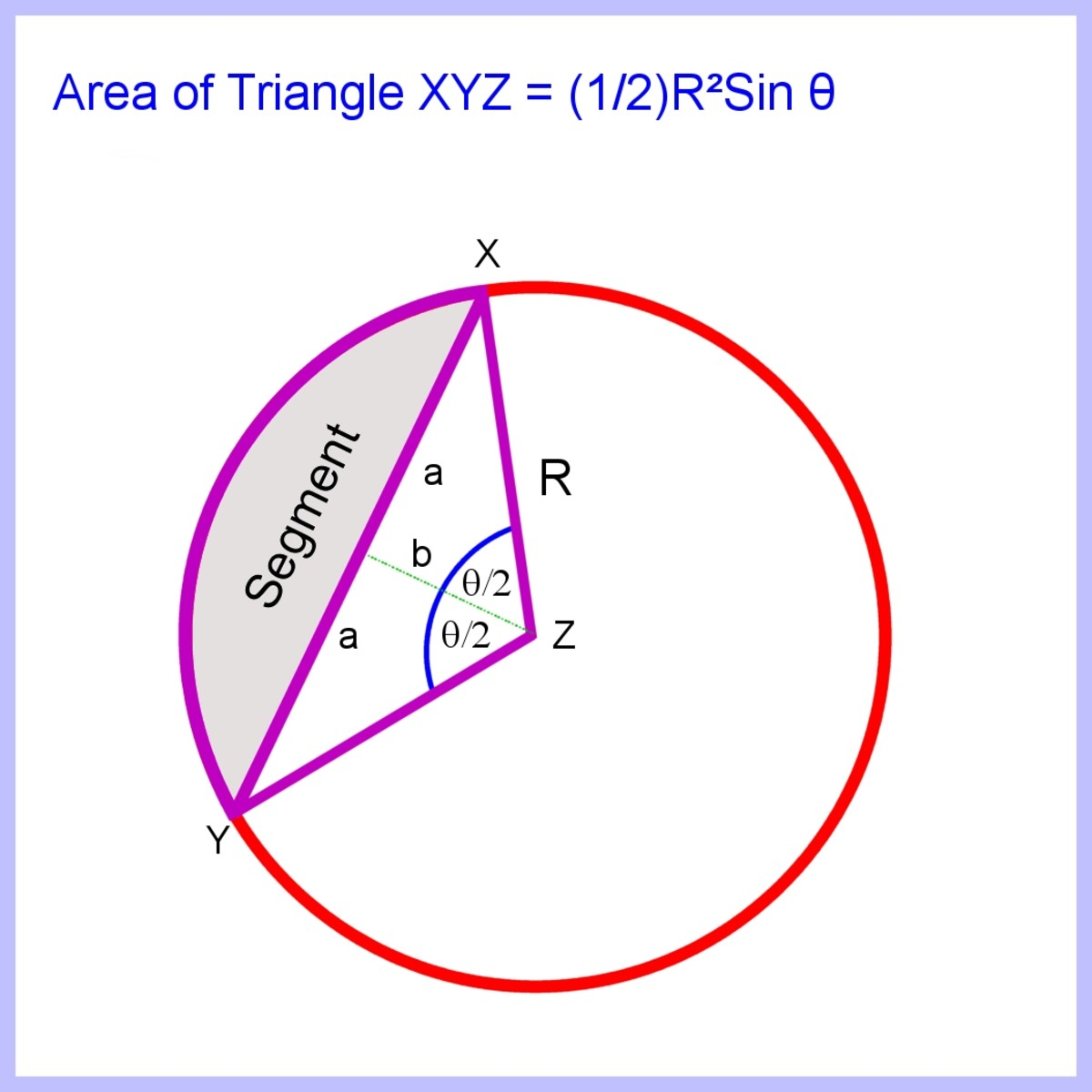To calculate the area of the segment, first calculate the area of the triangle XYZ and then subtract it from the sector.Image © Eugene Brennan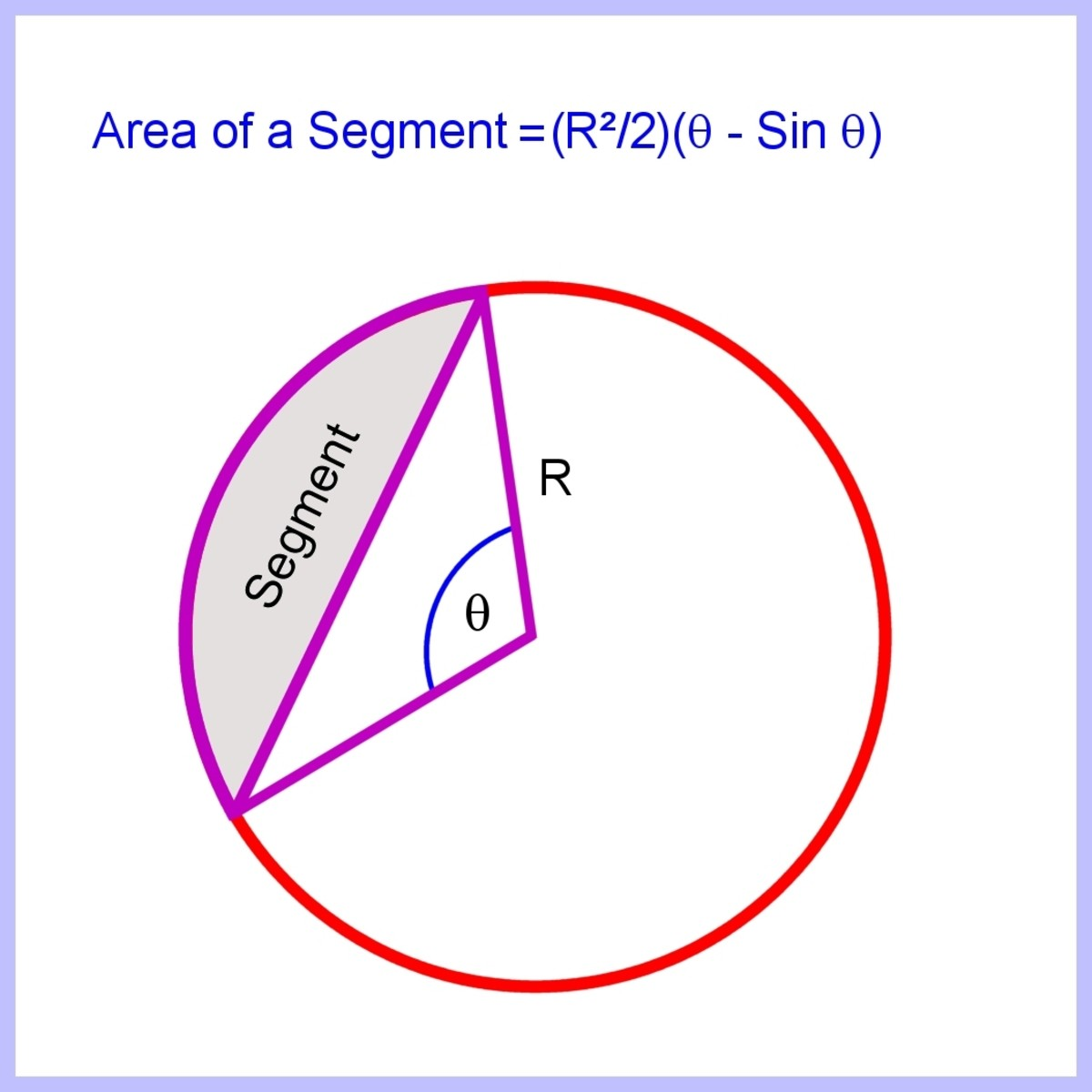Area of a segment of a circle knowing the angleImage © Eugene Brennan

## Equation of a Circle in Standard Form

If the centre of a circle is located at the origin, we can take any point on the circumference and superimpose a right angled triangle with the hypotenuse joining this point to the centre.
Then from Pythagoras's theorem, the square on the hypotenuse equals the sum of the squares on the other two sides. If the radius of a circle is r then this is the hypotenuse of the right angled triangle so we can write the equation as:

x2 + y2 = r2

This is the equation of a circle in standard form in Cartesian coordinates.

If the circle is centred at the point (a,b), the equation of the circle is:

(x - a)2 + (y - b)2 = r2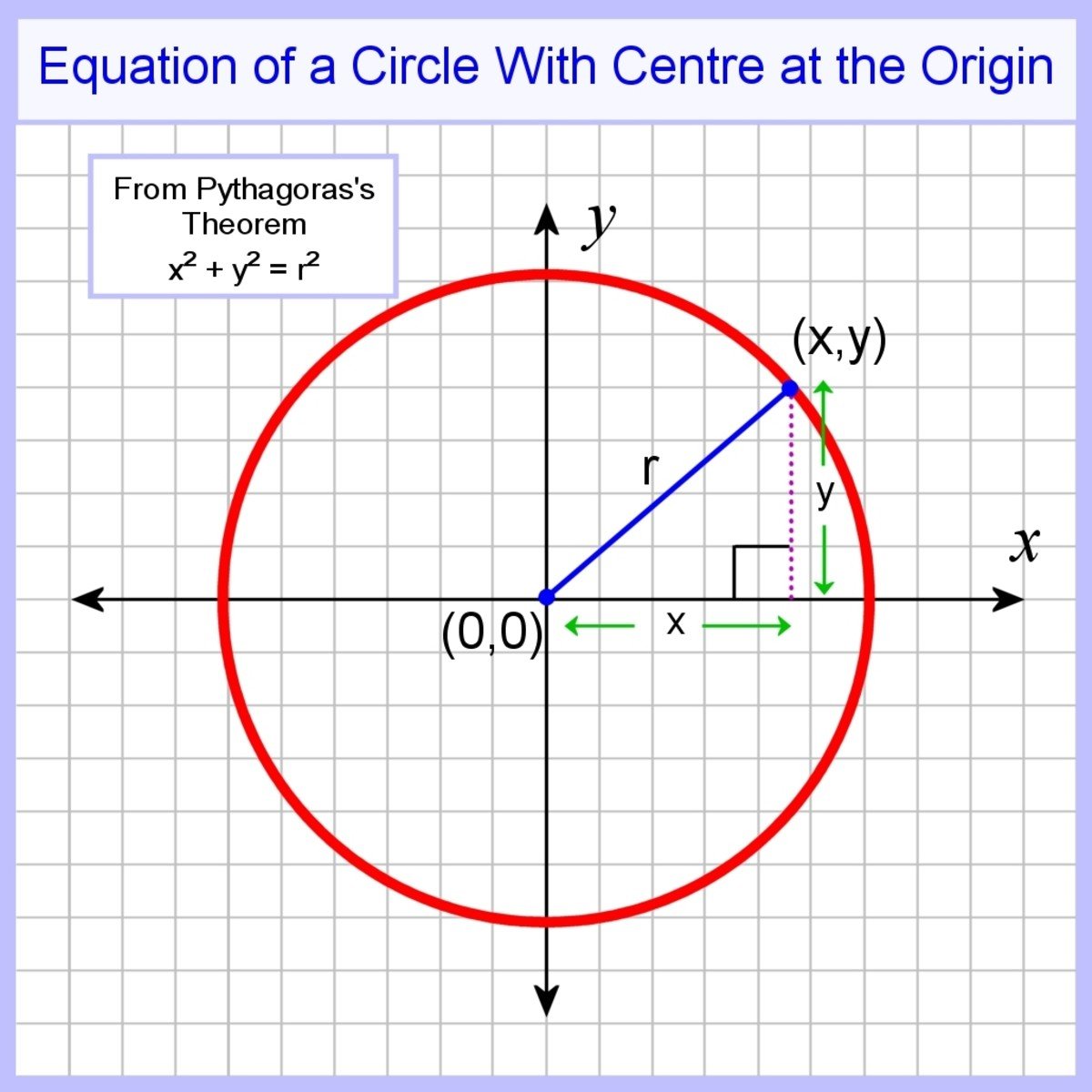The equation of a circle with a centre at the origin is r² = x² + y²Image © Eugene Brennan

## Equation of a Circle in Parametric Form

Another way of representing the coordinates of a circle is in parametric form. This expresses the values for the x and y coordinates in terms of a parameter. The parameter is chosen as the angle between the x-axis and the line joining the point (x,y) to the origin. If this angle is θ, then:

x = cos θ

y = sin θ

for 0 < θ < 360°

## Summary of Equations for a Circle

Circle formulas. θ is in radians.

QuantityEquation

Circumference

πD

Area

πR²

Arc Length

Chord Length

2Rsin(θ/2)

Sector Area

R²θ/2

Segment Area

(R²/2) (θ - sin(θ))

Perpendicular distance from circle centre to chord

Rcos(θ/2)

Angle subtended by arc

arc length / (Rθ)

Angle subtended by chord

2arcsin(chord length / (2R))

## Example

Here's a practical example of using trigonometry with arcs and chords. A curved wall is built in front of a building. The wall is a section of a circle. It's necessary to work out the distance from points on the curve to the wall of the building (distance "B"), knowing the radius of curvature R, chord length L, distance from chord to wall S and distance from centre line to point on curve A. See if you can determine how the equations were derived. Hint: Use Pythagoras's Theorem.

This article is accurate and true to the best of the author’s knowledge. Content is for informational or entertainment purposes only and does not substitute for personal counsel or professional advice in business, financial, legal, or technical matters.

© 2018 Eugene Brennan

Eugene Brennan (author) from Ireland on May 31, 2020:

Thanks Austen.

I worked out D in the diagram above knowing R and L/2. In reality that's probably not necessary because you may already know the distance from the centre of the arc to the inside of the wall. Adding this to S gives you D.

Austen on May 31, 2020:

Many thanks for solving the curved wall issue.

I’ racked my brains back 45 plus used the existing formulae on your website to crack it- but as always when someone who “knows” tells you “how” - it becomes so clear you wonder how you couldnt see it before.

Thanks for relighting the knowledge thirst.

A

Eugene Brennan (author) from Ireland on April 30, 2020:

Hi Austen, I spent hours trying to figure this out using angles, but it turned out that since the chord length is known between two ends of the curved wall (is this correct?), it can easily be worked out using Pythagoras's Theorem. I've drawn it up as an example at the bottom of the article, hope it helps.

Suggestion, you could put the values into a spreadsheet to do the calculations.

Austen SMITH on April 28, 2020:

Hi I have a simple but frustrating problem- I want to build a regular curved wall a set distance from a straight wall - the centre of the circle /arc of the wall falls within the building.

I need to work out distance from the straight wall to measure, at regular intervals, to create the perfect curve starting and ending on the chord (2nd) forming the distance from the straight wall.(1st chord)

Hope you can help.

Eugene Brennan (author) from Ireland on April 07, 2020:

If you mean the chord length, it's 2Rsin(θ/2).

See the derivation above.

darrell on April 06, 2020:

how do i calculate the length of a segment of a circle

Lakshay on September 19, 2019:

Good efforts

Eugene Brennan (author) from Ireland on April 05, 2019:

If you mean you know the coordinates of the start and end points of the chord, you can work out the length of the chord using Pythagoras's theorem. Then use the equation for length of a chord (2Rsin(θ/2) to find θ.

Mazin G A on April 01, 2019:

Hi,

How can I calculate the angle at the center of an arc knowing radius and center, start, and end points? I know how to do that if I have the length of the arc, but in my case I don't have it.

Eugene Brennan (author) from Ireland on March 19, 2019:

Thanks Troy, I'll keep it in mind. Parabolas will probably come first though.

Troy Sartain on March 19, 2019:

How about a similar article for ellipses? Just a thought. Obviously, another level of complexity, even if not rotated.

Larry Rankin from Oklahoma on May 19, 2018:

Very educational.

Eugene Brennan (author) from Ireland on May 18, 2018:

Thanks George, I should have proof read before publishing, instead of beta testing on the readers !!

George Dimitriadis from Templestowe on May 18, 2018:

Hi.

A good introduction to the basics of circle properties.

Diagrams are clear and informative.

Just a couple of points.

You have So C = πD = πR/2, which should be C = πD = 2πR

and A = πR^2 = π (D/2)2 = πD^2/2

should be A = πR^2 = π (D/2)2 = πD^2/4